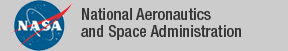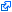### Top File of PVS complex Library

```
top: THEORY
%-------------------------------------------------------------------------------
% Complex Numbers
%
%     Author: David Lester, Manchester University & NIA
%
%
% The complex numbers are defined (axiomatically) in this way so that we can
% conveniently use the numeric constants 0, 1, 2 etc.
%
% The alternative -- using pairs of reals to represent the real and imaginary
% components -- would lead to the somewhat unappealing formulation of Euler's
% result as "exp((0,1)*(pi,0)) = (-1,0)". As a matter of taste, I prefer the
% somewhat more elegant formulation: "exp(i*pi) = -1".
%
% When theory interpretations can handle this, we'll be able to prove that the
% two formulations are equivalent, and hence use the beautiful version, and
% consigning the undignified version to the background!
%
% If we get really lucky, the number_fields_bis ("bis" from the Italian for
% extra) can be consigned to the dustbin of history, and it's functions
% incorporated into the general PVS decision procedures. In the mean time...
%
%
%     Version 1.0            5/29/04   Initial version (DRL)
%     Version 2.9            4/25/07
%------------------------------------------------------------------------------
BEGIN

IMPORTING complex_types,       % Basic Definitions of Complex Number Types
polar,               % Polar coordinate complex numbers
arithmetic,          % Basic Arithmetic on Complex Numbers
exp,                 % Complex logarithm and exponential functions
complex_sqrt,        % sqrt of a complex number
complex_field,       % complex numbers form a field
complex_sets         % set-valued complex functions

END top

```
The tagidentifies links that are outside the NASA domain